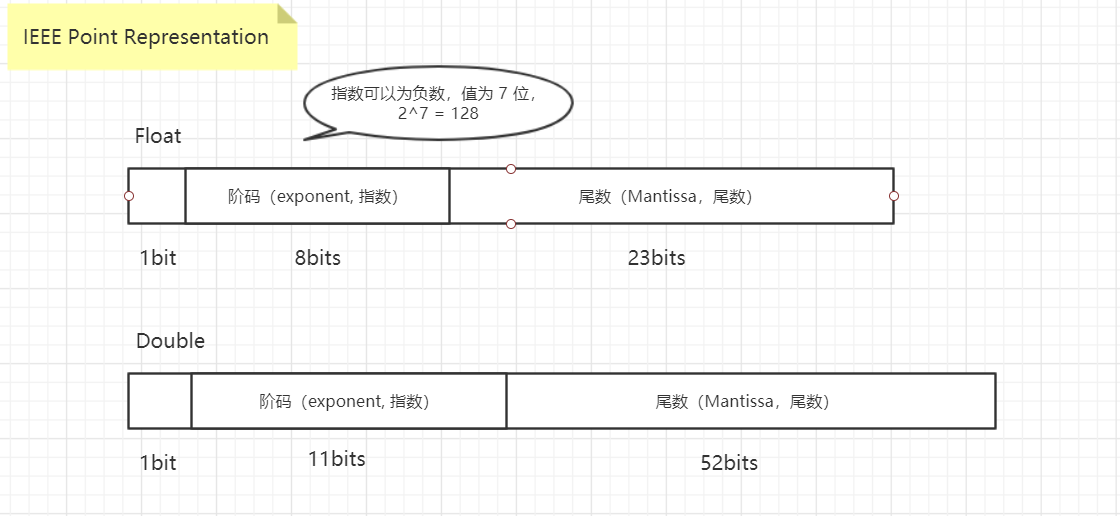# 不能使用 float 和 double 来表示金额等精确的值

### 不能使用 float 和 double 来表示金额等精确的值

Java 中 float 的精度为 6-7 位有效数字。double 的精度为 15-16 位，BigDecimal 用来对超过16位有效位的数进行精确的运算

#### 关于 float 和 double 的精度

float：2^23 = 8388608，一共七位尾数，这意味着最多能有 7 位有效数字，但绝对能保证的为6位，也即 float 的精度为 6~7 位有效数字；

double：2^52 = 4503599627370496，一共 16 位，同理，double 的精度为15~16位。

float 不是 4 个字节 32 位吗？为啥最大值是 2128-1（3.40E+38）呢？#### 问题一、出现的计算金额不准确，丢失精度：

public class Test {

public static void main(String[] args) {

double d1 = 11000;
double d2 = 0.35;
// 错误的：3849.9999999999995
System.out.println("错误的：" + d1 * d2);

BigDecimal bigDecimal1 = new BigDecimal(11000);
BigDecimal bigDecimal2 = BigDecimal.valueOf(0.35);
// multiply 乘法；正确的：3850.00
System.out.println("正确的：" + bigDecimal1.multiply(bigDecimal2));

}
}


#### 问题二、数据类型转换时会有问题

public class Test {

public static void main(String[] args) {
long longMaxVal = Long.MAX_VALUE;
double doubleVal = longMaxVal / 1.0;
double clone = doubleVal;

// 参考 Alibaba 《码出高效》, 两浮点数之差小于 diff 任务相等比较改进
float diff = 1e-6f;

System.out.println(doubleVal);
clone += 1000;
System.out.println(clone);

if (Math.abs(clone - doubleVal) < diff) {
// do
System.out.println("两数相等");
} else {
System.out.println("两数不相等");
}
}
}



0 个人打赏©️2019 CSDN 皮肤主题: 大白 设计师: CSDN官方博客